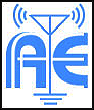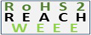Saturday, March 28, 2020 l 12:29:44 PM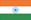Home Careers Enquiry Contact Us Site Map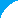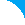OUR ESTEEMED CLIENTSHome » Knowledgebase » Technical Library

 Technical LibraryAntenna An antenna is a device to transmit and/or receive electromagnetic waves. Electromagnetic waves are often referred to as radio waves. Most antennas are resonant devices, which operate efficiently over a relatively narrow frequency band. An antenna must be tuned (matched) to the same frequency band as the radio system to which it is connected otherwise reception and/or transmission will be impaired. Wavelength We often refer to antenna size relative to wavelength. For example: a 1/2 wave dipole is approximately half a wavelength long. Wavelength is the distance a radio wave travels during one cycle. The formula for wavelength is λ = c / f where λ is the wavelength expressed in units of length, typically meters, feet or inches. Note: The physical length of a half-wave dipole is slightly less than a half-wavelength due to end effect. The speed of propagation in coaxial cable is slower than in air, so the wavelength in the cable is shorter. The velocity of propagation of electromagnetic waves in coax is usually given as a percentage of free space velocity, and is different for different types of coax. Impedance Matching For efficient transfer of energy, the impedance of the radio, the antenna and the transmission line connecting the radio to the antenna must be the same. Radios typically are designed for 50 Ohms impedance, and the coaxial cables (transmission lines) used with them also have a 50 Ohm impedance. Efficient antenna configurations often have an impedance other than 50 Ohms. Some sort of impedance matching circuit is then required to transform the antenna impedance to 50 Ohms. Antennas manufactured by Antenna experts come with the necessary impedance matching circuitry as part of the antenna. We use low loss-components in our matching circuits to provide the maximum transfer of energy between the transmission line and the antenna. VSWR and Reflected Power Voltage Standing Wave Ratio (VSWR) is an indication of the quality of the impedance match. VSWR is often abbreviated as SWR. A high VSWR is an indication the signal is reflected prior to being radiated by the antenna. VSWR and reflected power are different ways of measuring and expressing the same thing. A VSWR of 2.0:1 or less is often considered acceptable. Most commercial antennas, however, are specified to be 1.5:1 or less over some bandwidth. Based on a 100 watt radio, a 1.5:1 VSWR equates to a forward power of 96 watts and a reflected power of 4 watts, or the reflected power is 4.2% of the forward power. Bandwidth Bandwidth can be defined in terms of radiation patterns or VSWR/reflected power. The definition used is based on VSWR. Bandwidth is often expressed in terms of percent bandwidth, because the percent bandwidth is constant relative to frequency. If bandwidth is expressed in absolute units of frequency, for example MHz. Decibels Decibels (dB) are the accepted method of describing a gain or loss relationship in a communication system. The beauty of dB is they may be added and subtracted. A decibel relationship (for power) is calculated using the following formula dB = 10log(Pa/Pb). Pa might be the power applied to the connector on an antenna, the input terminal of an amplifier or one end of a transmission line. Pb might be the power arriving at the opposite end of the transmission line, the amplifier output or the peak power in the main lobe of radiated energy from an antenna. If Pa is larger than Pb the result will be a positive number or gain. If Pa is smaller than Pb the result will be a negative number or loss. Directivity and Gain Directivity is the ability of an antenna to focus energy in a particular direction when transmitting or to receive energy better from a particular direction when receiving. There is a relationship between gain and directivity. We see the phenomena of increased directivity when comparing a light bulb to a spotlight. A 100-watt spotlight will provide more light in a particular direction than a 100-watt light bulb and less light in other directions. We could say the spotlight has more "directivity" than the light bulb. The spotlight is comparable to an antenna with increased directivity. Gain is the practical value of the directivity. The relation between gain and directivity includes a new parameter η which describes the efficiency of the antenna as G = η x D. For example, an antenna with 3dB of directivity and 50% of efficiency will have a gain of 0 dB. Gain Measurement One method of measuring gain is to compare the antenna under test against a known standard antenna. This is known as a gain transfer technique. At lower frequencies, it is convenient to use a 1/2-wave dipole as the standard. At higher frequencies, it is common to use a calibrated gain horn as a gain standard with gain typically expressed in dBi. Another method for measuring gain is the 3-antenna method. Transmitted and received powers at the antenna terminal are measured between three arbitrary antennas at a known fixed distance. The Friis transmission formula is used to develop three equations and three unknowns. The equations are solved to find the gain expressed in dBi of all three antennas. Radiation Patterns Radiation or antenna pattern describes the relative strength of the radiated field in various directions from the antenna at a constant distance. The radiation pattern is a "reception pattern" as well, since it also describes the receiving properties of the antenna. The radiation pattern is three-dimensional, but it is difficult to display the three-dimensional radiation pattern in a meaningful manner. It is also time-consuming to measure a three-dimensional radiation pattern. Often radiation patterns measured are a slice of the three-dimensional pattern, resulting in a two-dimensional radiation pattern, which can be displayed easily on a screen or piece of paper. These pattern measurements are presented in either a rectangular or a polar format. Beamwidth Beamwidth describes the angular aperture where the most important part of the power is radiated. In general, we talk about the 3db beamwidth which represents the aperture (in degrees) where more than 90% of the energy is radiated. For example, for a 0 dB gain antenna, 3 db beamwidth is the area where the gain is higher than -3 dB. Near-Field and Far-Field Patterns The radiation pattern in the region close to the antenna is not exactly the same as the pattern at large distances. The term "near-field" refers to the field pattern existing close to the antenna; the term "far-field" refers to the field pattern at large distances. The far-field is also called the radiation field, and is what is most commonly of interest. The near-field is called the induction field (although it also has a radiation component). Ordinarily, it is the radiated power that is of interest so antenna patterns are usually measured in the far-field region. For pattern measurement it is important to choose a distance sufficiently large to be in the far-field, well out of the near-field. The minimum permissible distance depends on the dimensions of the antenna in relation to the wavelength. The accepted formula for this distance is rmin = 2D2 / λ where rmin is the minimum distance from the antenna; D is the largest dimension of the antenna; and λ is the wavelength. Antenna Polarization Polarization is defined as the orientation of the electric field of an electromagnetic wave. Two often-used special cases of elliptical polarization are linear polarization and circular polarization. The antenna launching the waves into space determines initial polarization of a radio wave. The environment through which the radio wave passes on its way from the transmit antenna to the receiving antenna may cause a change in polarization. With linear polarization the electric field vector stays in the same plane. In circular polarization the electric field vector appears to be rotating with circular motion about the direction of propagation, making one full turn for each RF cycle. The rotation may be right-hand or left-hand.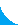*  info@antennaexperts.in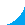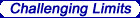© 2020 Antenna Experts. All Rights Reserved. This site is best viewed in 1024 x 768 resolution and above in Google Chrome Designed & Maintained by Net-the-Net (India)